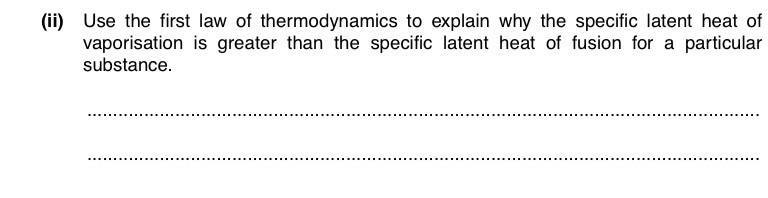# Why specific latent heat of vaporisation > fusion?

1. Homework Statement## Homework Equations

First law of thermodynamics, ΔU=q+W

## The Attempt at a Solution

Ok so, I know that when liquid evaporates, the change in volume is much greater than that when solid melts. And for both cases, distance of separation of atoms increases too, so PE increases, and hence, internal energy increases.
So, ΔU=mL+ pΔV
L=(ΔU-pΔV) /m
But how do we know that the term (ΔU-pΔV) is greater for vaporisation than that of fusion?

Ok so, I know that when liquid evaporates, the change in volume is much greater than that when solid melts.
Well, that is in fact the main essence of the answer.

So, ΔU=mL+ pΔV
There is a sign problem here. The work done on the system ##W## should be ## - p \Delta V## - do you see why?

Well, that is in fact the main essence of the answer.

There is a sign problem here. The work done on the system ##W## should be ## - p \Delta V## - do you see why?
Ahh I see why now. Yup, because it's work done by system.
Thanks !